Question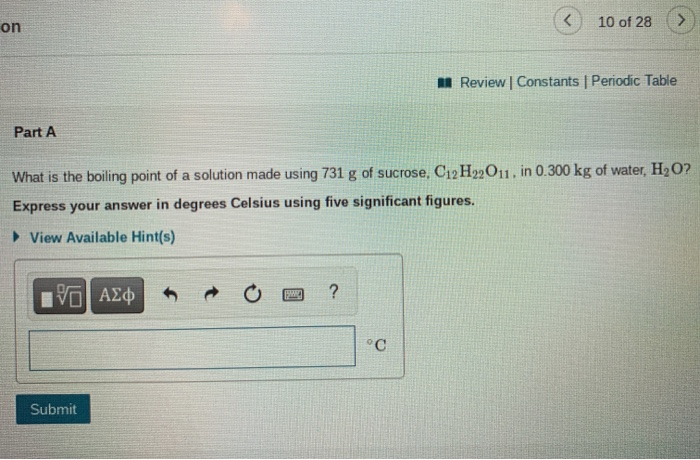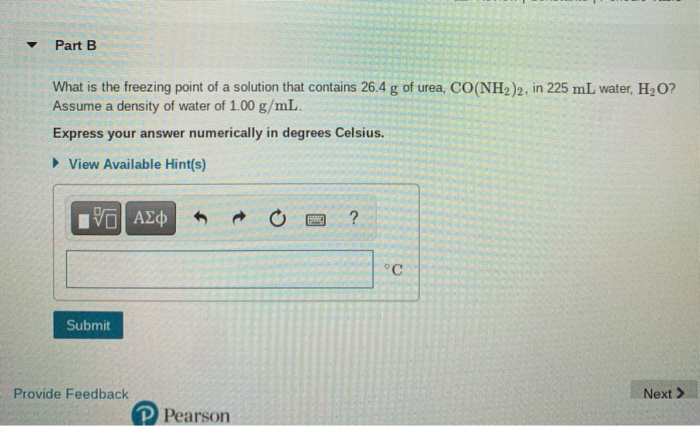We need at least 10 more requests to produce the answer.

0 / 10 have requested this problem solution

The more requests, the faster the answer.

All students who have requested the answer will be notified once they are available.

#### Earn Coins

Coins can be redeemed for fabulous gifts.

Similar Homework Help Questions
• ### Review Constants Periodic Table The changes in boiling point (AT) or freezing point (AT) in degrees...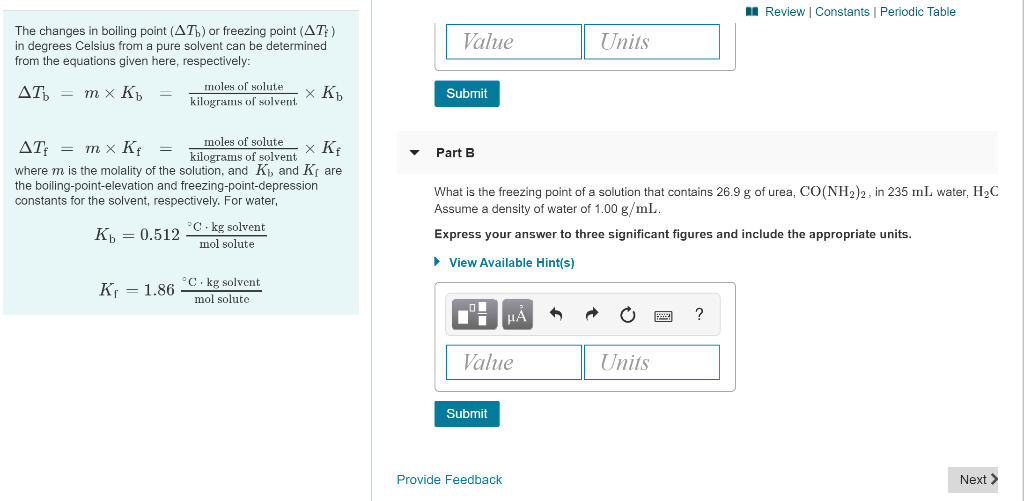Review Constants Periodic Table The changes in boiling point (AT) or freezing point (AT) in degrees Celsius from a pure solvent can be determined from the equations given here, respectively: Value Units moles of solute AT = mx Kb = 7 Submit kilograms of solvent XRb moles of solutex Kf Part B AT: = mx Kf = kilograms of solvent where m is the molality of the solution, and K and K the boiling-point-elevation and freezing-point-depression constants for the solvent,...

• ### A Review Constants Periodic Table The changes in boiling point (AT) or freezing point (AT) in...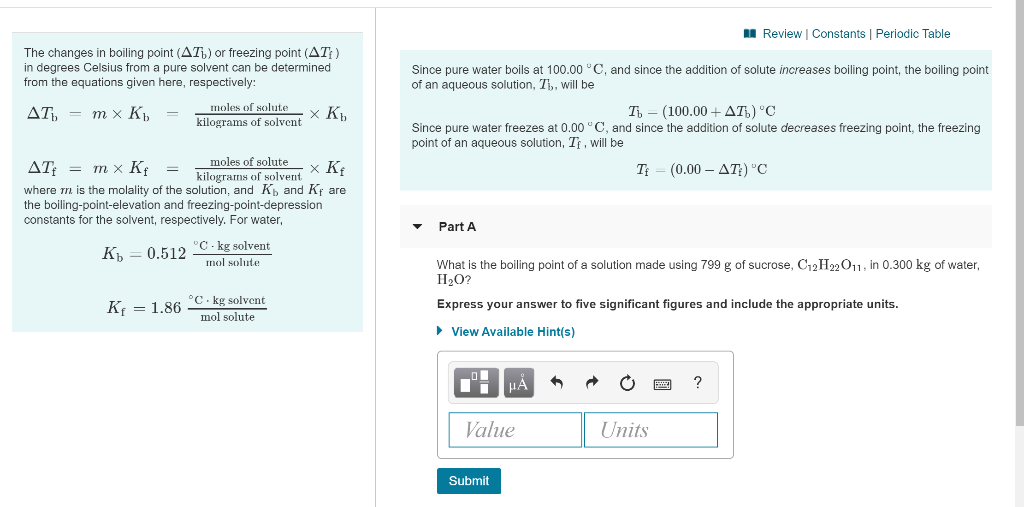A Review Constants Periodic Table The changes in boiling point (AT) or freezing point (AT) in degrees Celsius from a pure solvent can be determined from the equations given here, respectively: AT) = m x K = moles of solute XK K. kilograms of solvent Since pure water boils at 100.00 °C, and since the addition of solute increases boiling point, the boiling point of an aqueous solution, Th, will be T - (100.00+AT) 'C Since pure water freezes at...

• ### 3 of 28 > A Review Constants Periodic Table Part A You have prepared a saturated...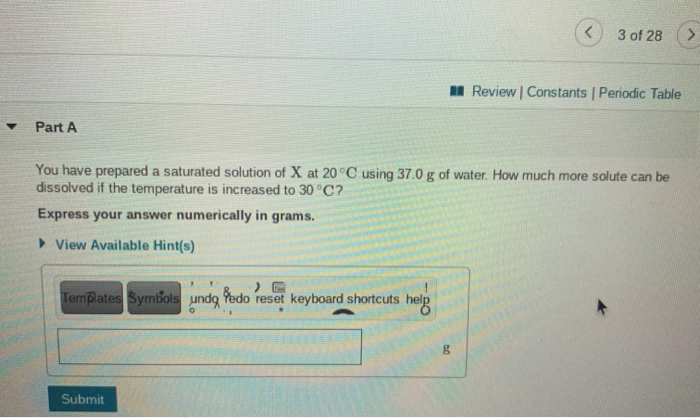3 of 28 > A Review Constants Periodic Table Part A You have prepared a saturated solution of X at 20°C using 37.0 g of water. How much more solute can be dissolved if the temperature is increased to 30°C? Express your answer numerically in grams. View Available Hint(s) Templates Symbols undg Pedo reset keyboard shortcuts help R B Submit < 4 of 28 M Review Constants Periodic Table Part A Calculate the mass percent of a solution that is...

• ### 2559b3823f31 > 8 of 28 A Review Constants Periodic Table ✓ Correct Part B What is...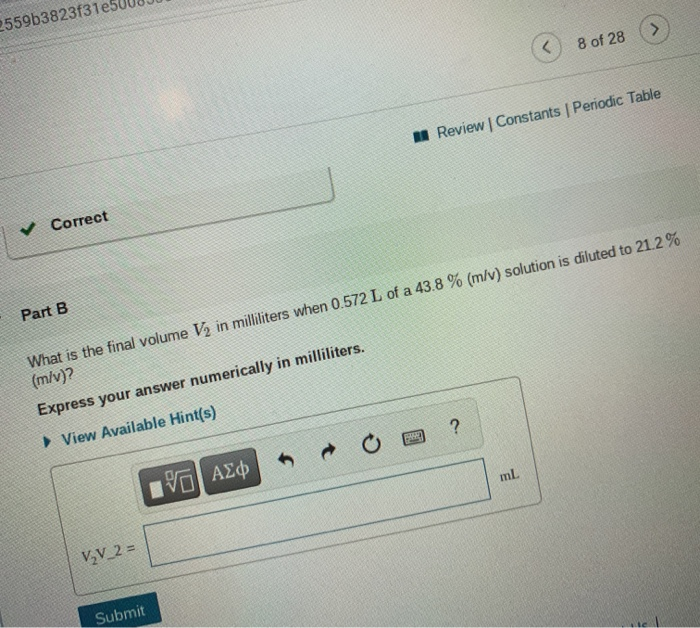2559b3823f31 > 8 of 28 A Review Constants Periodic Table ✓ Correct Part B What is the final volume V2 in milliliters when 0.572 L of a 43.8 % (m/v) solution is diluted to 21.2% (m/v)? Express your answer numerically in milliliters. View Available Hint(s) ? ml IVO AXO V₂V_2= Submit 8 of 28 M Review | Constants Periodic Table Part C A 554 ml NaCl solution is diluted to a volume of 1.08 L and a concentration of 2.00...

• ### 9 of 28 A Review Constants | Periodic Table 2HCl(aq) + Na2CO3(aq) +2NaCl(aq) + H2O(l) +...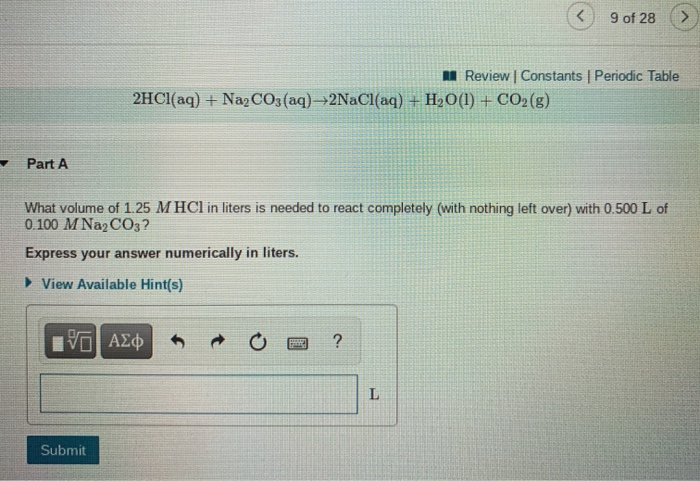9 of 28 A Review Constants | Periodic Table 2HCl(aq) + Na2CO3(aq) +2NaCl(aq) + H2O(l) + CO2(g) Part A What volume of 1.25 M HCl in liters is needed to react completely (with nothing left over) with 0.500 L of 0.100 M Na2CO3? Express your answer numerically in liters. ► View Available Hint(s) ΟΙ ΑΣΦ ? L Submit 9 of 28 Review | Constants Periodic Table Part B A 381-mL sample of unknown HCl solution reacts completely with Na2CO3 to...

• ### Review Constants Periodic Table Part A A stainless steel alloy is to be analyzed for its...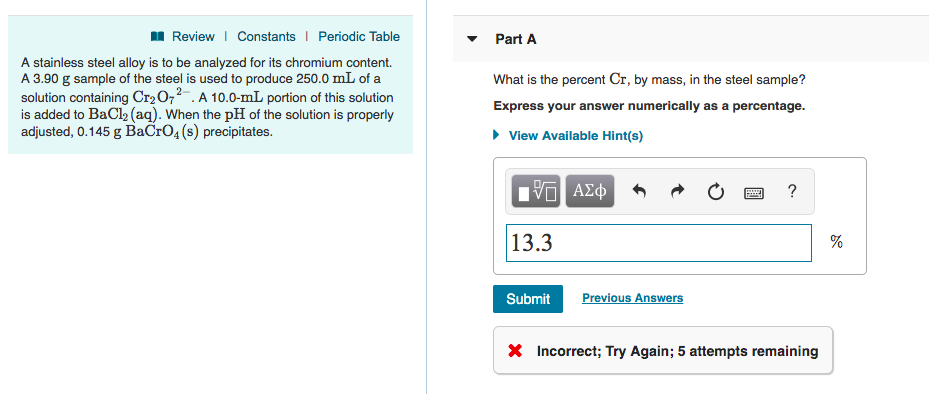Review Constants Periodic Table Part A A stainless steel alloy is to be analyzed for its chromium content A 3.90 g sample of the steel is used to produce 250.0 mL of a solution containing Cr2O72.A 10.0-mL portion of this solution is added to BaCl2 (aq). When the pH of the solution is properly adjusted, 0.145 g BaCrO4 (s) precipitates. What is the percent Cr, by mass, in the steel sample? Express your answer numerically as a percentage. View Available...

• ### 27 of 28 Review | Constants penVellumHMAC=056d4e636002559b3823831e5008538910001 с Part A 19 AZ Calculate the freezing point...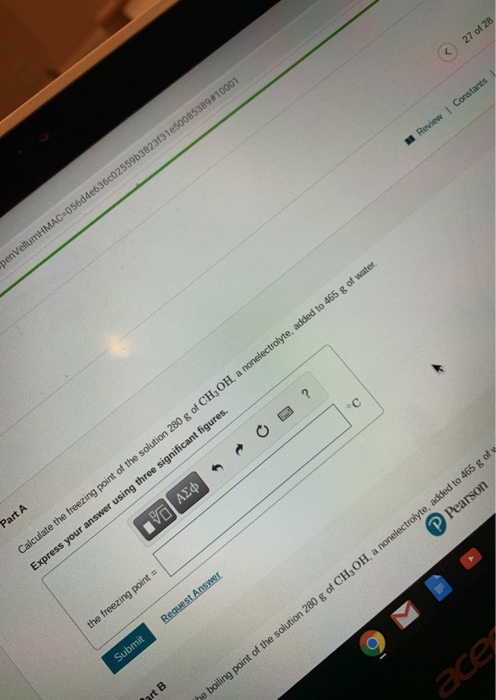27 of 28 Review | Constants penVellumHMAC=056d4e636002559b3823831e5008538910001 с Part A 19 AZ Calculate the freezing point of the solution 280 g of CH3OH, a nonelectrolyte, added to 465 g of water. Express your answer using three significant figures. Request Answer the freezing point = Submit ace art B he boiling point of the solution 280 g of CH, OH, a nonelectrolyte, added to 465 g of Pearson Part B Calculate the boiling point of the solution 280 g of CH3OH,...

• ### The Arrhenius Equation 11 of 25 Part A Review Constants Periodic Table 30.3 kJ/mol At 20...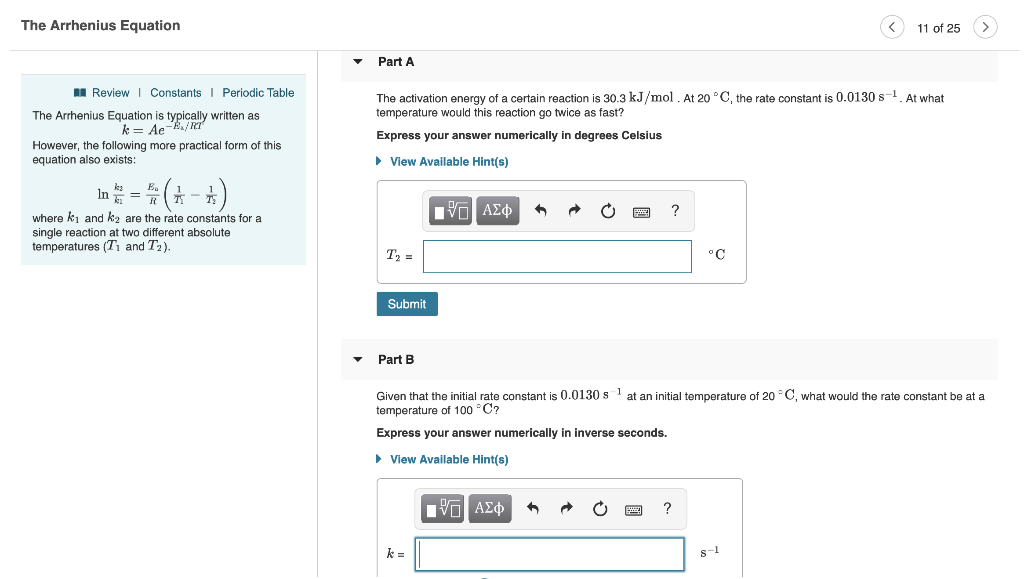The Arrhenius Equation 11 of 25 Part A Review Constants Periodic Table 30.3 kJ/mol At 20 C, the rate constant is 0.0130 s. At what The activation energy of a certain reaction temperature would this reaction go twice as fa The Arrhenius Equation is typically written as k Ae more practical form of this Express your answer numerically in degrees Celsius However, the foll equation also existe View Available Hint(s) ln ΑΣφ where ki and k2 are the rate constants...

• ### < 9 of 13 Review Constants Periodic Table Part A A calorimeter contains 28.0 mL of...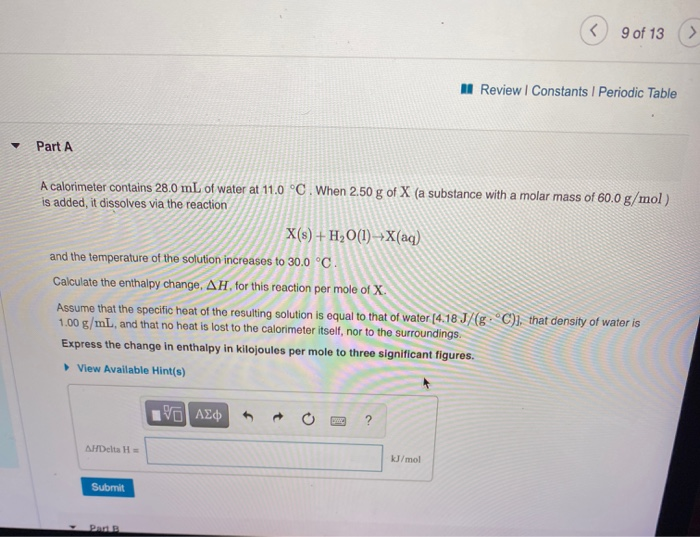< 9 of 13 Review Constants Periodic Table Part A A calorimeter contains 28.0 mL of water at 11.0 °C. When 250 g of X (a substance with a molar mass of 60.0 g/mol) is added, it dissolves via the reaction X(s) + H2O(1) X(aq) and the temperature of the solution increases to 30.0 °C. Calculate the enthalpy change, AH, for this reaction per mole of X. Assume that the specific heat of the resulting solution is equal to that...

• ### < 9 of 13 Review Constants Periodic Table Part A A calorimeter contains 28.0 mL of...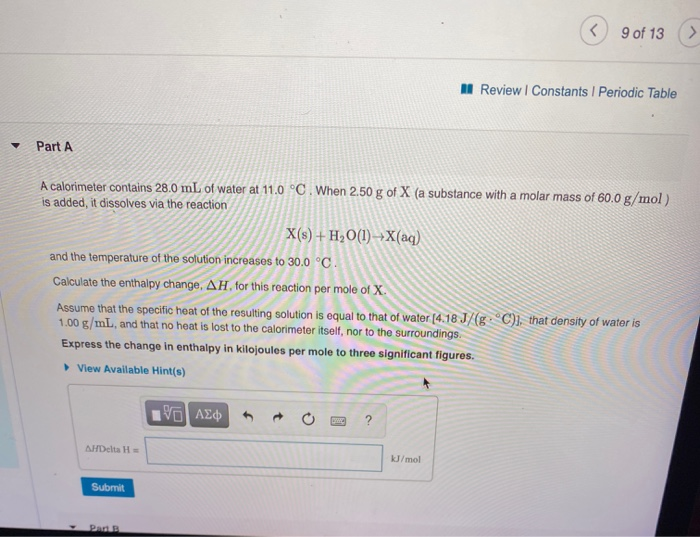< 9 of 13 Review Constants Periodic Table Part A A calorimeter contains 28.0 mL of water at 11.0 °C. When 250 g of X (a substance with a molar mass of 60.0 g/mol) is added, it dissolves via the reaction X(s) + H2O(1) X(aq) and the temperature of the solution increases to 30.0 °C. Calculate the enthalpy change, AH, for this reaction per mole of X. Assume that the specific heat of the resulting solution is equal to that...